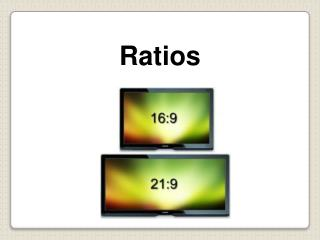DownloadDownload PresentationRatios

Ratios

Télécharger la présentationRatios

- - - - - - - - - - - - - - - - - - - - - - - - - - - E N D - - - - - - - - - - - - - - - - - - - - - - - - - - -
Presentation Transcript

1. Ratios

2. Ratio is a way of comparing two or more quantities • Examples: • Number of oranges to apples • Number of girls in a class compared to boys Ratio?

3. There are 3 ways to express ratios: As a fraction - ¾ Using a colon - 3:4 Using the word “to” - 3 to 4

4. What is the ratio of cats to mice? 3 Number of Cats: 6 Number of Mice: Express the ratio as a fraction: 3 to 6 Express the ratio in words: Express the ratio with a colon: 3:6

5. 2001 Spring Training List the wins to losses by the Cubs : 17 to 14 17 to 31 Wins to games played by the Cubs: List the losses to wins by the Padres: 11/16 Losses to games played by the Padres : 11/27

6. 2001 Spring Training List the Dodgers wins to total games: 18 : 31 List the Dodgers losses to wins: 13 : 18

7. Your face uses about 13 muscles to smile and about 43 muscles to frown. Write the ratio of muscles used for frowning to muscles used for smiling in three different ways. 43:13 43/13 43 to 13

8. Comparing Ratios Seth and Nathan compared their CD collections. To determine who has a greater ratio of rock CDs to pop CDs, write the ratios. Nathan has a greater ratio of rock to pop CDs. Seth: = .375 Write ratios as fractions. Nathan: = 0.6 Write fractions as decimals to compare.

9. How do you change a fraction into a decimal? Divide the denominator into the numerator OR on your calculator hit 9 divided by 24 = .375

10. Ratio Example Problems RED & BLUESmarties Blue : Red 6 : 3 Which symplifies to 2 : 1 6 BLUE 3 RED “The Ratio of BLUE to RED Smarties is 6 to 3 “The Ratio of BLUE to REDSmarties is 6

11. RED & BLUESmarties Red : Blue 3 : 6 “For every RED Smartie, there are 2 BLUES” 1 : 2 6 BLUE 3 RED …. Or… We can compare Red to Blue Smarties! “The Ratio of RED to BLUESmarties is 1:2” “The Ratio of RED to BLUE Smarties is 3 to 6”

12. In the Smarties example, we changed 6:3 to 2:1 • This is called Simplifying a ratio • We did it by dividing each number by 3 • 3 was the largest common factor Simplifying Ratios

13. 8:2 18:3 4:8 5:25 5:2 4:1 6:1 1:2 1:5 5:2 !! Try to simplify these

14. “Bart and Maggie share a bag of 16 life savers with the ratio of 3:1” This means “for every 3 life savers Bart gets, Maggie gets 1” Sharing an amount in a given ratio 4 Lifesavers for Maggie 12 Lifesavers for Bart

15. What is a ratio? Example: There are 300 computers and 1200 students in our school. What is the ratio of computers to students? A ratio is a comparison of two quantities. Express the ratio in words: 300 computers to 1200 students Express the ratio with a colon: 300 computers : 1200 students Express the ratio as a fraction: How many students are there for one computer?

16. What is an Equivalent Ratio? 1.There are 300 computers for 1200 students OR 2.There is 1 computer for every 4 students Express the ratio as a fraction: Equivalent ratiosmake the same comparison. Bring the fraction to lowest terms:

17. Practice With Equivalent Ratios Find an equivalent ratio by dividing: # 1  Divide by 30 # 2  Divide by 3 # 3  Divide by 25

18. Practice With Equivalent Ratios Find an equivalent ratio by multiplying: # 4  Multiply by 5 # 5  Multiply by 12 # 6  Multiply by 6

19. Practice:Ratios worksheet